RUSSIAN JOURNAL OF EARTH SCIENCES, VOL. 19, ES6001, doi:10.2205/2019ES000687, 2019

# Analogy method to determine the stress-strain state of structural-tectonic blocks of the Earth's crust for the disposal of radioactive waste

V. N. Morozov1, V. N. Tatarinov1,2, A. I. Manevich1,3, I. V. Losev1,3

1Geophysical Center RAS, Moscow, Russia

2Schmidt Institute of Physics of the Earth RAS, Moscow, Russia

3Mining Institute, NUST "MISiS", Moscow, Russia

### Abstract

The authors have analyzed the influence of tectonic faults located in the external stress field on the process of destruction of structural tectonic blocks. The article describes the impact of changes in the regional field of tectonic stresses on the geoecological safety of a deep radioactive waste disposal facility in the Nizhnekansky massif (the Krasnoyarsk Territory). The authors compared the results of modeling the stress-strain state of conditionally equivalent environment for two hierarchical levels: destruction of tile cladding of buildings and underground structures and carried out this comparison on a mega scale, for the epicentral zones of crust earthquakes. The above results provide a basis for using the analogy method for predicting the stability of structural tectonic blocks, since the obtained qualitative regularities of the process of destruction of the geological environment are not sensitive to the scale effect.

### 1. Introduction

When solving complex geological problems, one often has to resort to "non-strict" means of spatial and temporal extrapolations, based on logical conclusions and analogies, "the knowledge of certain principles easily compensates the lack of knowledge of certain facts" (Claude Adrien Helvétius). Such technique is called an "analogy method".

Analogy method, as an integral part of system analysis, is widely used in geomechanics, mining, geoinformatics and other domains of Earth sciences as a method of spatio-temporal extrapolation of knowledge about the geological environment [Chernykh, 2013; Gvishiani et al., 2018; Nazarov, 1982, et al.]. In mining, the choice of development systems for mineral deposits and their parameters is based on world experience in establishing similar deposits in similar mining and geological conditions. The accumulated experience and its application to the analog object increases the efficiency of exploration work to optimize the conditions for mining deposits.

There are multiple uncertainties related to the problem of underground isolation of long-lived radioactive waste (RW), especially when substantiating the stability of the structural-tectonic block (STB), which contains a deep radioactive waste disposal facility (RWDF), for a period of its radiobiological hazard exceeding 10 thousand years. We can solve this problem by applying the analogy method. The stability of STB implies its ability under the certain geological and tectonic conditions to maintain its integrity and insulating properties within the time limits admissible for the possible migration of radionuclides during the entire operation of RWDF [Gvishiani et al., 2019].

The analogy method involves the implementation of a systematic sequence of cognitive procedures. At the first stage, the requirements for the proposed prototype are formulated and the purpose of the analogue search process is determined. The second stage is the search and selection of a prototype that would most fully meet the conditions of the task. Next, a system of similar parameters is established for the object and prototype. At this stage, the corresponding parameters of the objects are compared in terms of the number of similar and different features and their significance. The next stage is a transfer (extrapolation) of information from the studied objects to unknown ones, conclusion, and inference, which is the key part of the method, determining its main character [Nazarov, 1982].

Developing the idea of M. V. Gzowski on the fundamental possibility of modeling the development of tectonic processes in space and time [Gzovsky, 1975], the study is based on the method of mathematical modeling of the stress-strain state (SSS) of the medium. The essence of the method is described in detail in [Morozov et al., 2008, 2012; et al.]. For simulation we used a model of block heterogeneous elastic-isotropic medium disturbed by a system of tectonic faults. The elastic moduli of faults are substantially lower than the elastic moduli of the surrounding geological environment. Fault tectonics in zones of active orogenesis (including the Nizhnekansky massif) is a determining factor in predicting the long-term stability of STB intended for RW disposal. Ranking STB according to the degree of concentration of tectonic stresses (elastic strain energy) is a logically justified concept in the framework of continuum fracture mechanics [Morozov et al., 2011], but at the same time it does not exclude the need for experimental confirmation. This is primarily due to the physical and mathematical model of the geological environment (a layer in a plane stress state - 2D model) and the boundary conditions. The experimental confirmation also fully relates to the elastic parameters of tectonic faults. In the models, faults are represented as a dispersed medium with zones of influence in the form of leading echelon cracks [Anderson et al., 2011].

The following are examples of comparing the modeling of SSS of a layer broken by discontinuities (aggregate-filled cracks) with the results of mathematical modeling of the stress-strain state of conditionally equivalent environment at the micro level (destruction of tiles in the cladding of buildings and underground structures) and on a megascale in the epicentral zones of crustal earthquakes.

Since the mathematical model of SSS is not sensitive to the scale effect, the results can be the basis for the analogy method for predicting the stability of STBs located in the field of tectonic stresses.

### 2. Method of Stress-Strain State Modeling

The works previously published [Morozov et al., 2008, 2011, 2012] describe the method for modeling SSS of block heterogeneous massifs disturbed by tectonic faults. Briefly, it includes the following: a block heterogeneous rock mass, consisting of elastic-isotropic blocks, is modeled by a layer that is broken by randomly oriented faults. In this case, faults are subvertical. By tectonic faults we mean a dispersed medium ("giant block rock melange") [Kocharyan, 2016; Sherman et al., 1992; Zabrodin et al., 2015]). The width of the zone of dynamic influence of the fault is accepted within 1 km. The elastic modulus of rocks of the fault zone is two orders of magnitude lower than those of the surrounding massif [Morozov and Manevich, 2016; Morozov et al., 2008].

The authors used a finite element model of the geological medium located in the external field of tectonic stresses (in terms of a plane stress state). The relations between stresses and strains are taken averaged over the thickness of the layer, according to the model of a generalized plane stress state of Hooke's law (1):

\begin{equation} \tag*{(1)} \left\{ \begin{array}{lll} \sigma_{xx} \sigma_{yy} \sigma_{xy} \end{array} \right\} = \bigl[ D(E^{(m)}, \nu^{(m)}) \bigr] \times \left\{ \begin{array}{lll} \varepsilon_{xx} \varepsilon_{yy} \varepsilon_{xy} \end{array} \right\} \end{equation}

where $\sigma_{xx}$, $\sigma_{yy}$, $\sigma_{xy}$ are the components of averaged integrated stresses; $\varepsilon_{xx}$, $\varepsilon_{yy}$, $\varepsilon_{xy}$ are the corresponding components of strain tensor; $E^{(m)}$ – the Young's modulus; $V^{(m)}$ – poisson's ratio of the elasticity matrix of a single finite element, with the help of which inhomogeneity (fracture) is introduced into the elastic-isotropic model of the layer by the formula (2):

\begin{eqnarray*} \bigl[ D(E^{(m)}, \nu^{(m)}) \bigr] = E^{(m)} \times \bigl[ 1- (\nu^{(m)})^2 \bigr] \times \end{eqnarray*}

\begin{equation} \tag*{(2)} \left[ \begin{array}{ccc} 1 & \nu^{(m)} & 0 \nu^{(m)} & 1 & 0 0 & 0 & (1 - \nu^{(m)})^2 \end{array} \right] \end{equation}

In addition to the stress tensor components $\sigma_{xx}$, $\sigma_{yy}$, $\sigma_{xy}$, the stress intensity was calculated by the formula (3):

\begin{equation} \tag*{(3)} \sigma_i = (\sigma_{xx}^2 + \sigma_{yy}^2 -\sigma_{xx} \times \sigma_{yy} + 3 \times \sigma_{xy}^2 )^{1/2} \end{equation}

Stress intensity is an indicator of the energy saturation of a fragment of the geological environment, since the potential energy of shape change is determined by the formula (4):

\begin{equation} \tag*{(4)} U_{\Phi} = \frac{1 + \bar{\nu}}{3 \times \bar{E}} \times \sigma_i^2 \Delta V \end{equation}

where $\bar E$ and $\bar \nu$ are average elastic moduli, $\Delta V$ is volume.

At that, the response stress corresponds to the Dinnick concept [Dinnick, 1946]:

\begin{eqnarray*} \sigma_{xx} = \frac{\nu}{1 - \nu} \sigma_{yy} \end{eqnarray*}

where $\nu$ is the Poisson's ratio.

The subject of analysis is the stress intensity $\sigma_i$, stress tensor components $\sigma_{xx}$, $\sigma_{yy}$, $\sigma_{xy}$, as well as the ratio of the principal stresses $\sigma_{yy}/ \sigma_{xx}$. For all calculations, we used the assumed numerical parameters of the operating compressive tectonic stresses (their orientation is shown on the calculation schemes) $\sigma_{\max} = 30$ MPa, $\sigma_{\min} = 10$ MPa according to the results of measurements of the main stresses in the upper part of the Earth's crust in various regions of the globe [Zubkov, 2016]. The value of the elastic modulus of the surrounding geological environment is assumed to be $E = 5 \times 10^4$ MPa, the modulus of the dispersed fault material is two orders of magnitude lower, $E_{\mathrm{disp}} = 5 \times 10^2$ MPa, Poisson's ratio $\nu = 0.25$.

### 3. Comparison of the Results of Modeling SSS at a Micro Level

Tiles made from geomaterial (granite, marble etc.) and synthetic ceramic tiles (including crushed rock) are widely used for construction purposes when working on building facades and interior spaces, including underground structures (metro stations etc.). For such kind of works cement mixtures are used, to fill in the space between wall and tiles.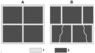Figure 1

During construction process, two types of tiles are used (Figure 1). It was found that fracture and crack formation most frequently occurs in the second type of cladding (b) in the corners of tiles, i.e. in areas of local stress concentration.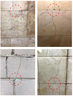Figure 2

This effect was found on tiles of different sizes (from $50 \times 50$ cm to $10 \times 10$ cm) and thickness both on the facades of buildings and at metro stations (Figure 2). In contrast to extended cracks (bulging cracks) during deformation of a significant fragment of a building structure, these foci of destruction are local in nature. Figure 2 shows photographs of examples of this type of destruction of tiles.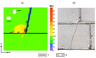Figure 3

Thus, we can assume that the tile layer with underlying cement partially perceives the gravitational load of the weight of the building structure, which, taking into account the time factor, leads to a local stress concentration and crack formation. Moreover, the crack propagates in the background stress field, that is, the accumulated strain energy in the local zone is realized in the form of a propagating crack and beyond it. The results of modeling the stress-strain state in these areas are shown in Figure 3a.

The localization area of high stress intensity at the junction of three tiles causes the formation of a crack and its development in the background stress field. Considering the obtained result as modeling SSS of a block medium on a laboratory scale, we can conclude that the results of modeling SSS at a laboratory analogue give an explanation for the appearance of local fields of stress concentration and subsequent crack formation (on a mega-fault scale). Considering the results as a laboratory experiment, i.e. modeling a block massif with faults as an analogue of modeling a seismotectonic process on rock samples, we analyze the SSS models in the epicentral zones of strong crustal earthquakes in seismically active regions in the next section.

### 4. Results of Numerical Simulation of SSS of Epicentral Area of the Crust Earthquakes

As is known, tectonic faults are the result of tectonic stresses in the upper part of the Earth's crust. Fault parameters (length, orientation, angle of incidence etc.) and tectonic fracture zones are the criteria for determining the orientation of the main tectonic stresses in the studied region. The absolute value of the current tectonic stresses is determined by seismological data and instrumental measurements in mine workings and wells [Zubkov, 2016]. The presence of local heterogeneity and tectonic faults in the medium leads to a local concentration of tectonic stresses, significantly exceeding the background stress values.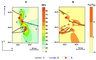Figure 4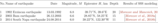Table 1

As we have shown previously, the local concentration of tectonic stresses in the epicentral zones of strong crustal earthquakes determined the position of the fault and its length, and therefore the possible energy of the earthquake [Morozov and Manevich, 2016, 2018; Morozov et al., 2018a, 2018b]. As an example, we consider the stress-strain state of three crustal earthquakes with $M > 6$ and focal depths ranging from 10 to 20 km (Table 1). The Table 1, Figure 4 and Figure 6 show the parameters of earthquakes and calculated values of the stress intensity (according to (4), the square of the stress intensity is proportional to the stored strain energy) and the main stress ratio $\sigma_{yy}/\sigma_{xx}$.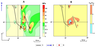Figure 5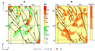Figure 6

In the figures (Figure 4, Figure 5, and Figure 6), the local stress concentration arises due to the presence of faults. Asterisks indicate the epicenters of strong earthquakes localized in zones of high stress intensity, that is, the maximum potential strain energy preceding the formation of a fault. Moreover, the epicenter of the earthquake (the projection of the source to the surface) is in the region of maximum values of the ratio of the main acting stresses $\sigma_{yy}/\sigma_{xx}$. The given examples provide grounds to assert that the used geomechanical model retrospectively adequately reflects the SSS of the epicentral zones of crustal earthquakes, with the subsequent formation of a fault during the main seismic event. This model can be used as a predictive criterion for the stability of STB in the external field of tectonic stresses.

### 5. Results of Numerical Simulation of SSS of Nizhnekansky Granitoid Massif

The works [Anderson et al., 2011; Belov et al., 2007; Ozersky, 2012; Kochkin, 2019] emphasized the ambiguity of structural tectonic schemes of the Nizhnekansky massif (NKM). Fault tectonics, which is a fundamental factor in assessing the stability of STB in the field of tectonic stresses, has never been studied with a sufficient degree of reliability. Geophysical studies often do not confirm both the interpretation of satellite images and tectonic faults. In this regard, the structural-tectonic scheme used in the northern part of the Nizhnekansky massif should be considered as a methodological basis for calculating SSS and assessing the stability of RWDF site.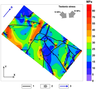Figure 7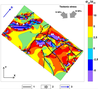Figure 8

Thus, using the analogy method and bearing in mind the epicentral zones of strong crustal earthquakes as analogous objects, we can assume that the stress concentration zones are dangerous for RWDF. Therefore, a low level of stress concentration in comparison with background stresses and the ratio $\sigma_{yy}/\sigma_{xx}$ can be used as a criterion for the stability of STB when choosing the location of RWDF. Figure 7Figure 8 show the results of modeling SSS of the Nizhnekansky massif area, namely, stress intensity $\sigma_i$ maps and stress ratios $\sigma_{yy}/\sigma_{xx}$. For the simulation we used a computational structural-tectonic scheme, compiled from the works [Anderson et al., 2011]. The accepted differentiation of the elastic moduli of faults and rocks of the massif causes a local stress concentration (concentration of elastic strain energy), which can cause the appearance of new tectonic disturbances in the creep or seismic activity mode.

It can be seen that in the external field of tectonic stresses, zones of anomalously high stress intensity arise $(\sigma_i > 25$ MPa). Drawing an analogy with the results of modeling the SSS of the earthquake zones of the previous section, the gap appeared at the periphery of zones with anomalously high stress intensities $\sigma_i$ and low compression stresses $\sigma_{xx}$, that transform into tensile stresses. The analogy method in this case gives reason to believe that the destruction of the rock mass most likely occurs under the similar geomechanical conditions. Comparison of stress intensity maps within the NKM (subject to the reliability of the structural-tectonic scheme) allows us to identify the zone of possible formation of a tectonic fault.

As in the epicentral zones of strong crustal earthquakes given in the previous section, a high concentration of stress intensity ($\sim 30$ MPA) with the ratio $\sigma_{yy}/\sigma_{xx} > 3$ (Figure 8, dashed line), in the zone of possible fault formation, this section can be excluded from the area of possible RWDF site. And similarly, we recommend safer places within areas with low tectonic stress.

### 6. Summary

Conclusions about the possible tectonic destruction of structural-tectonic blocks of the Earth's crust in the areas of underground isolation of highly active RW, obtained by analogy method, have a high degree of reliability. System-oriented approaches make possible more reliable intertemporal and spatial extrapolations of our knowledge of tectonic processes.

Setting up an underground research laboratory within the Nizhnekansky granitoid massif in order to assess the suitability of the selected site for underground isolation of highly active long-lived RW opens up new possibilities in studying the development of tectonic process in this area, from the point of view of assessing the reliability of forecast estimates of stability of structural-tectonic blocks as independent elements. Combining instrumental observations on the Earth's surface with the deformations of the Earth's crust and the behavior of marginal zones of mine workings at a depth of 500 meters will allow us to obtain comparable data on the reaction of the rock mass to the external field of tectonic stresses, and, accordingly, to evaluate the preservation of the natural insulating properties of the geological environment.

Modeling SSS of a rock mass makes it possible to detect zones of high stress intensity that are dangerous from the standpoint of the probable tectonic destruction of STB. The analogy method to analyze the results of stress modeling is an interpretative prerequisite for predicting the probability of formation of a fault crossing the RW deep burial site. This is due to a change in the hydrogeological conditions of the facility and the danger of the unintended spread of radionuclides in the environment.

It is shown that areas of low stress intensity are promising from the point of view of safer disposal of radioactive waste from the standpoint of tectonic stability of the geological environment. The above results are useful for the development of methods to predict the stability of structural-tectonic blocks of the Earth's crust for the disposal of RW and to carry out complex geophysical research in an underground research laboratory within the Nizhnekansky massif.

#### Acknowledgments

The research was supported by Russian science foundation grant (proj. \numero 18-17-00241).

### References

Anderson, E. B., S. V. Belov, E. N. Kamnev, I. Yu. Kolesnikov, N. F. Lobanov, V. N. Morozov, V. N. Tatarinov (2011) , Underground Isolation of Radioactive Waste, 592 pp., Mining Book, Moscow.

Belov, S. V., V. N. Morozov, V. N. Tatarinov, E. N. Kamnev, J. Hammer (2007) , Study of the structure and geodynamic evolution of the Nizhnekansky Massif in connection with the disposal of highly radioactive waste, Geoecology, no. 2, p. 248–266.

Chernykh, V. V. (2013) , Actualism and the principle of analogies in geological retrospectives, Lithosphere, no. 4, p. 49–39.

Dinnick, A. N. (1946) , Stability of Arches, 127 pp., OGIZ GOSTEHIZDAT, Moscow.

Gvishiani, A. D., L. A. Weisberg, V. N. Tatarinov, A. I. Manevich (2018) , System analysis in mining sciences and decreasing environment damage, Proceedings of the conference dedicated to the memory of Academician A. V. Kryazhimsky "System Analysis: Modeling and Management", p. 43–45, Institute of Mathematics named V. A. Steklov, RAS, Moscow, https://doi.org/10.4213/proc20588.

Gvishiani, A. D., V. I. Kaftan, R. I. Krasnoperov, V. N. Tatarinov (2019) , Geoinformatics and systems analysis in geophysics and geodynamic, Earth Physics, no. 1, p. 42–60, https://doi.org/10.31857/S0002-33372019142-60.

Gzovsky, M. V. (1975) , Basics of Tectonophysics, 536 pp., Science, Moscow.

Kocharyan, G. G. (2016) , Geomechanics of Faults, 424 pp., GEOS, Moscow.

Kochkin, B. T. (2019) , Investigating the geological environment at the Yeniseisky site: tasks for the current stage of the disposal project, Radioactive Waste, 2, no. 7, p. 76–91, https://doi.org/10 25283/2587-9707-2019-2-76-91.

Morozov, V. N., A. I. Manevich (2016) , Modeling stress-strain state in the epicentral zone of the earthquake 26.01.2001, $M=6.9$ (India), Geophysical Research, 17, no. 4, p. 23–36, https://doi.org/10.21455/gr2016.4-2.

Morozov, V. N., A. I. Manevich (2018) , Modeling stress-strain state in the epicentral zone of the earthquake 13.03.1992, $Ms = 6.8$ (Turkey), Geophysical Research, 19, no. 1, p. 17–29, https://doi.org/10.21455/gr2018.1-2.

Morozov, V. N., I. Yu. Kolesnikov, S. V. Belov, V. N. Tatarinov (2008) , Stress-strain state Nizhnekansky massif – the area of possible disposal of radioactive waste, Geoecology, no. 3, p. 232–243.

Morozov, V. N., I. Yu. Kolesnikov, V. N. Tatarinov (2011) , Modeling of danger levels of stress-strain state in structural blocks of the Nizhnekansky granitoid massif (to the choice of radioactive waste disposal sites), Geoecology, no. 6, p. 524–542.

Morozov, V. N., I. Yu. Kolesnikov, V. N. Tatarinov (2012) , Modeling the Hazard Levels of Stress-Strain State in Structural Blocks in Nizhnekanskii Granitoid Massif for Selecting Nuclear Waste Disposal Sites, Water Resources, 39, no. 7, p. 756–769, https://doi.org/10.1134/S009780781207007X.

Morozov, V. N., V. N. Tatarinov, I. Yu. Kolesnikov, A. I. Manevich (2018) , Modeling the Stress-Strain State in the Epicentral Zone of a Strong Earthquake in Iran (December 26, 2003, $Mw = 6.6$), Izvestiya, Physics of the Solid Earth, 54, no. 4, p. 602–611, https://doi.org/10.1134/S1069351318040080.

Morozov, V. N., V. I. Kaftan, V. N. Tatarinov, I. Yu. Kolesnikov, A. I. Manevich, A. Yu. Melnikov (2018) , Numerical Modeling of the Stress-Strain State and Results of GPS Monitoring of the Epicentral Area of the August 24, 2014 Earthquake (Napa, California, USA), Geotectonics, 52, no. 5, p. 578–588, https://doi.org/10.1134/S0016852118040064.

Nazarov, I. V. (1982) , Methodology of Geological Research, 177 pp., Science, Novosibirsk.

Ozersky, A. Yu. (2012) , Geochemical peculiarities of the Lower archean rocks at the site of probable underground construction in the southern part of the Yenisei Ridge, Exploration and Protection of Mineral Resources, 7, p. 39–44.

Sherman, S. I., et al. (1992) , Faulting in the Lithosphere: Shear Zones, 258 pp., Nauka, Novosibirsk.

Zabrodin, V. Yu., O. V. Ryas, G. Z. Gilmanova (2015) , Fault Tectonics of the Russian Far East Mainland, 132 pp., Dal'nauka, Vladivostok.

Zubkov, A. V. (2016) , The low of natural stress formation of the Earth's crust, Lithosphere, no. 5, p. 146–151.

Received 25 September 2019; accepted 18 October 2019; published 12 November 2019.Citation: Morozov V. N., V. N. Tatarinov, A. I. Manevich, I. V. Losev (2019), Analogy method to determine the stress-strain state of structural-tectonic blocks of the Earth's crust for the disposal of radioactive waste, Russ. J. Earth Sci., 19, ES6001, doi:10.2205/2019ES000687.

Generated from LaTeX source by ELXfinal, v.2.0 software package.